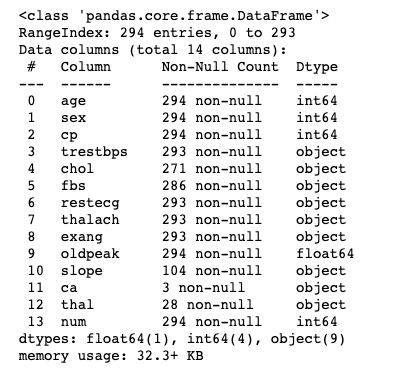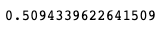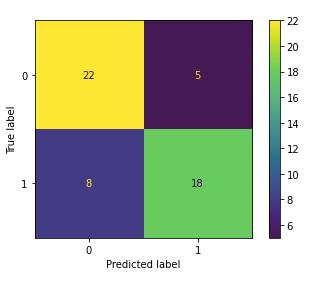top of page
Search
•Magdalena Konkiewicz

# Build your first random forest classifier and predict heart disease across patientsImage by Gordon Johnson from Pixabay

Introduction

In this post, I will guide you through building a simple classifier using Random Forest from the scikit-learn library.

We will start by downloading data set from Kaggle, after that, we will do some basic data cleaning, and finally, we will fit the model and evaluate it. On the way, we will also create a baseline model that will be used for evaluation.

We will be working with Heart Disease Data set that can be downloaded from Kaggle using this link.

This data set consists of almost 300 hundred patients that either have or do not have heart issues. This is what we will be predicting.

In order to do this, we will use thirteen different features:

1. age

2. sex

3. chest pain type (4 values)

4. resting blood pressure

5. serum cholesterol in mg/dl

6. fasting blood sugar > 120 mg/dl

7. resting electrocardiographic results (values 0,1,2)

8. maximum heart rate achieved

9. exercise induced angina

10. oldpeak = ST depression induced by exercise relative to rest

11. the slope of the peak exercise ST segment

12. number of major vessels (0-3) colored by fluoroscopy

13. thal: 3 = normal; 6 = fixed defect; 7 = reversable defect

Take time to familiarize yourself with these descriptions now so you have an understanding of what each column represents.

Once you have downloaded the data set and placed it in the same folder as your Jupyter notebook file, you can use the following commands to load the data set.

```
import pandas as pd

```This is the head of the data frame that you will be working with.

Data Cleaning

Did you spot question marks in the data frame above? It looks like the author of this data set have used them to indicate null values. Let's replace them with real Nones.

```
df.replace({'?': None}, inplace=True)

```

Now that we have done that we can inspect how many null values are in our data set. We can do this with info() function.

```
df.info()

```We can see here that columns 10, 11, and 12 have a lot of nulls. 'Ca' and 'thal' are actually almost empty and 'slope' has only 104 entries. This is too many missing values to fill in so let's drop them.

```
df.drop(columns=['slope', 'thal', 'ca'], inplace=True)

```

The rest of the columns have none or little missing values. For simplicity, I suggest to drop the entries that do have them. We should not lose too much data.

```
df.dropna(inplace=True)

```

Another information that we could read from the result of the info() function is the fact that most of the columns are objects even though they seem to have numeric values.

My suspicion is that this was caused by the question marks in the initial data set. Now that we have removed them we should be able to change the objects to numeric values.

In order to do this, we will use pd.to_numeric() function on the whole data frame. The object values should become numbers and it should not affect the values that already numbers.

```
df = df.apply(pd.to_numeric)
df.info()

```As you can see we are now left only with floats and integers. The info() function also confirm that the columns 'Ca', 'thal', and 'slope' were dropped.

Also, rows with null values got removed and as a result, we have a data set with 261 numeric variables.

There is one more thing we need to do before we can proceed. I have noticed that the last column 'num' has some trailing spaces in its name (you cannot see this with a bare eye) so let's have a look at the list of column names.

```
df.columns

```You should see the trailing spaces in the last column 'num'. Let's remove them by applying strip() function.

```
df.columns = [column.strip() for column in df.columns]

```Done!

Exploratory Data Analysis

Let's do some basic data analysis. We are going to look at the distribution of variables using histograms first.

```
import matplotlib.pyplot as plt
plt.figure(figsize=(10,10))
df.hist()
plt.tight_layout()

```What we can notice straight away is the fact that some variables are not continuous. Actually, only five features are continuous:''age', 'chol', 'oldpeak', 'thalach', 'trestbps' whereas the other are categorical variables.

Because we want to treat them differently in our exploration we should divide them into two groups.

```
continous_features = ['age', 'chol', 'oldpeak', 'thalach', 'trestbps']

non_continous_features = list(set(df.columns) - set(continous_features + ['num']))

```

After doing this you can check their values by typing the variable names in Jupyter notebook cell.

```
continous_features``````
non_continous_features```Now we would like to inspect how the continuous features differ across the target variable. We will do this with a scatterplot.

```
import seaborn as sns
df.num = df.num.map({0: 'no', 1: 'yes'})
sns.pairplot(df[continous_features + ['num']], hue='num')

```* Note that we had to make the 'num' variable a string in order to use it as a hue parameter. We did it by mapping 0s to 'no' meaning healthy patients, and 1s to 'yes' meaning patients with heart disease.

If you look at the scatterplots and kdes you can see that there are district patterns for patients with heart disease in comparison to patients who are healthy.

In order to explore categorical variables, we will look at distinct values they can take by using describe() function.

```
df[non_continous_features].applymap(str).describe()

```We can see that 'exang', 'fbs' and 'sex' are binary (they take only two distinct values). Whereas 'cp' and 'resteceg' take respectively four and three distinct values.

The last two are ordered categorical variables as encoded by the data set authors. I am not sure if we should treat them like that or change them to dummy encodings. This would need further investigation and we could change the approach in the future. For now, we will leave them ordered.

Last but not least we are going to explore the target variable.

```
df.num.value_counts()```We have 163 healthy patients and 98 patients with heart problems. Not ideally balanced data set but that should be ok for our purposes.

Creating a baseline model

After a quick exploratory data analysis, we are ready to build an initial classifier. We are going to start by dividing the data set into features and the target variable.

```
X = df.drop(columns='num')
y = df.num.map({'no': 0, 'yes': 1})

```

* Note that I have to reverse the mapping I have applied while creating a seaborn graph, therefore, a need for map() function while creating y variable.

We also have used all features that the data set had as by looking at our quick EDA they all seemed relevant.

Now we will divide X and y variables further into their train and test correspondents using train_test_split() function.

```
from sklearn.model_selection import train_test_split

X_train, X_test, y_train, y_test = train_test_split(X, y, test_size=0.2, random_state=1)

X_train.shape, X_test.shape, y_train.shape, y_test.shape

```As a result of the above operations, we should have now four different variables: X_train, X_test, y_train, y_test whose dimensions are printed above.

Now we will build a baseline using a DummyClassifier.

```
from sklearn.dummy import DummyClassifier
from sklearn.metrics import accuracy_score
dc = DummyClassifier(strategy='most_frequent')
dc.fit(X,y)
dc_preds = dc.predict(X)
accuracy_score(y, dc_preds)

```As you can see the baseline classifier is giving us 62% accuracy on the train set. The strategy for our baseline is predicting the most frequent class.

Let's see if we can beat it with Random Forest.

Random Forest Classifier

The code below sets a Random Forest Classifier and uses cross-validation to see how well it performs on different folds.

```
from sklearn.ensemble import RandomForestClassifier
from sklearn.model_selection import cross_val_score

rfc = RandomForestClassifier(n_estimators=100, random_state=1)
cross_val_score(rfc, X, y, cv=5)

```As you can see these accuracies are in general much higher than our dummy baseline. Only the last fold has lower accuracy. It looks like this last fold has examples that are hard to recognize.

Nevertheless, if we take the average of those five, we get an accuracy of around 74%, and this is much higher than 62% baseline.

Normally this is a stage where we would like to further tune model parameters using for example GridSearchCV but this is not a part of this tutorial.

Let's see how well the model performs on the test set now. If you have paid attention we have not done anything with the test so far. It has been left alone until now.

Evaluating the model

We will start by checking model performance in terms of accuracy.

First, we will fit the model using the whole training data, and then we will call the accuracy_score() function on the test parts.

```
rfc.fit(X_train, y_train)
accuracy_score(rfc.predict(X_test), y_test)

```We are getting 75% accuracy on the test. Similar to our average cross-validation accuracy calculation on the train set which was 74%.

Let's see how well the Dummy classifier does on the test set.

```
accuracy_score(dc.predict(X_test), y_test)

```Accuracy for the baseline classifier is around 51%. This is actually much worse than the accuracy of our random forest model.

However, we should not only look at accuracy when evaluating a classifier. Let's have a looks at confusion matrices for both random forest and the baseline model.

We will start with computing confusion matrix for Random Forest using scikit-learn function.

```
from sklearn.metrics import plot_confusion_matrix
plot_confusion_matrix(rfc, X_test, y_test)

```Actually we are not doing bad at all. We only have five False Positives, and also eight False Negatives. Additionally, we have predicted heart disease for eighteen people out of twenty-six people that had heart problems.

Not great but not that bad. Note that we did not even tune the model!

Let's compare this confusion matrix with the one calculated for the baseline model.

```
from sklearn.metrics import plot_confusion_matrix
plot_confusion_matrix(dc, X_test, y_test)

```Have a closer look at the graph above. Can you see that we always predict label 0? This means we predict that all patients are healthy!

That is right, we have set our Dummy Classifier to predict the majority class. Note that it would be a terrible model for our purposes as we would not discover any patients with heart issues.

Random Forest did much better! We actually have discovered 18 people with heart problems out of 26 in the test set.

Summary

In this post, you have learned how to build a basic classifier using Random Forest.

It was rather an overview of the main techniques that are used when building a model on a data set without going into too many details.

This was intended so this article does not get too long and serves as a starting point for someone who wants to build their first classifier.

Happy Learning!